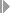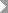Show TOC

###(02) Revenue-Based Method Without Profit Realization#### Use

You can use the Revenue-Based Method Without Profit Realization for:

• Sales orders

• Projects

• Internal orders with revenue, service orders with revenue, maintenance orders with revenue

Revenue-based results analysis without profit realization does not calculate a profit as long as the actual revenue is less than the planned cost.

Revenue-based results analysis without profit realization allows you to:

• Create reserves for unrealized costs

• Create reserves for imminent losses

• Use milestone billing

The system only reports a profit if the profit has been realized on the market.

Revenue-based results analysis without profit realization allows you to follow conservative balance sheet guidelines.

#### Prerequisites

You have planned costs and revenues.

Choose a results analysis method in simplified Customizing for Product Cost by Sales Order underPeriod-End ClosingResults AnalysisValuation Method.

#### Features

POC = R(a) / R(p)

R(PA) = R(a)

If R(a) < C(p) then C(PA) = R(a)

If C(p) <= R(a) < R(p) then C(PA) = C(p)

If R(a) >= R(p) then C(PA) = POC * C(p) = R(a) / R(p) * C(p)

The revenue affecting net income equals the actual revenue. It can be transferred to CO-PA together with the cost of sales.

If the actual costs are greater than the costs affecting net income, the system creates capitalized costs.

If the actual costs are less than the costs affecting net income, the system creates reserves for unrealized costs.

Inventory values and reserves for unrealized costs can be transferred to FI and EC-PCA when you settle.

The cost of sales is zero as long as your actual revenue is zero. The capitalized costs are then equal to the actual costs. Earnings are only calculated when revenues have been received.

If actual revenue is less than planned cost, the system sets the costs affecting net income to be the same as the revenue affecting net income in the amount of the actual revenue. In this case no profit is calculated. Capitalized costs and reserves can be reported.

#### Example

You have planned revenues of USD 200,000 and costs of USD 120,000 for your sales order.

Period 01

In period 01 you have actual costs of USD 20,000 but no revenues. In results analysis, the system calculates the following data:

• Capitalized costs in the amount of the actual costs (USD 20,000)

• Revenue of USD 0

• Cost of sales of USD 0

• You then settle the following:

• The capitalized costs to FI and EC-PCA

• No cost of sales and no revenues to CO-PA

The following values are reported in CO-PA:

Profitability Analysis

 Revenues 0 Cost of sales 0 Profit 0

The income statement shows the following values:

Income Statement

 Expense Revenue Actual costs 20,000 Inventory increase capitalized costs 20,000 20,000 20,000

Period 02

In period 02 the actual costs increase by USD 60,000 and now total USD 80,000. You deliver to your customer and send him a milestone invoice for USD 100,000. The order is partially delivered and partially billed. In results analysis, the system calculates the following data:

• Cost of sales of USD 100,000

Since the actual revenue is less than the planned costs, the cost of sales is equal to the actual revenue.

• Reserves for unrealized costs of USD 20,000 using the formula: Reserves for Unrealized Costs = Costs Affecting Net Income – Actual Costs if Actual Costs < Costs Affecting Net Income. The capitalized costs are canceled.

• Revenues of USD 100,000

You then settle the following:

• The cost of sales to CO-PA

• The revenues to CO-PA

• The reserves for unrealized costs and the cancellation of the capitalized costs to FI and EC-PCA

The following values are reported in CO-PA:

Profitability Analysis

 Revenues 100,000 Cost of sales 100,000 Profit 0

The income statement shows the following values:

Income Statement

 Expense Revenue Actual costs 80,000 Actual revenue 100,000 Reserves for unrealized costs 20,000 Profit 0 100,000 100,000

In contrast to the revenue-based method with profit realization , this profit has not been realized on the market. If you are using the revenue-based method with profit realization, the system reports an income surplus in Financial Accounting of USD 40,000 at this point.

Period 03

In period 03 the actual costs increase by USD 10,000 and now total USD 90,000. You deliver a second amount to your customer and send him a second milestone billing for USD 90,000. The total revenue is USD 190,000. The order is partially delivered and partially billed. In results analysis, the system calculates the following data:

• Cost of sales of USD 120,000 using the formula: Costs Affecting Net Income = Planned Costs

• Reserves for unrealized costs of USD 30,000 using the formula: Reserves for Unrealized Costs = Costs Affecting Net Income – Actual Costs if Actual Costs < Costs Affecting Net Income

• Revenue affecting net income of USD 190,000

You then settle the following:

• The change in the cost of sales to CO-PA

• The change in the revenues to CO-PA

• The change in the reserves for unrealized costs to FI and EC-PCA

The following values are reported in CO-PA:

Profitability Analysis

 Revenues 190,000 Cost of sales 120,000 Profit 70,000

The income statement shows the following values:

Income Statement

 Expense Revenue Actual costs 90,000 Actual revenue 190,000 Reserves for unrealized costs 30,000 Profit 70,000 190,000 190,000

In contrast to period 02, in period 03 a profit is reported. This is because the actual revenues now exceed the planned costs.

Period 04

In period 04 the actual costs increase by USD 40,000 and now total USD 130,000. You deliver the remaining goods and send the customer the final invoice for USD 10,000. The total revenue is USD 200,000. The order is now fully delivered and fully invoiced.

In results analysis, the system calculates the following:

• Cost of sales of USD 130,000

Since the actual costs exceed the planned costs, the system sets the cost of sales to the value of the actual costs.

• No reserves for unrealized costs. The existing reserves are canceled.

• Revenues of USD 200,000

You then settle the following:

• The change in the cost of sales to CO-PA

• The change in the revenues to CO-PA

• The cancellation of the reserves to FI and EC-PCA

The following values are reported in CO-PA:

Profitability Analysis

 Revenues 200,000 Cost of sales 130,000 Profit 70,000

The income statement shows the following values:

Income Statement

 Expense Revenue Actual costs 130,000 Actual revenue 200,000 Profit 70,000 200,000 200,000

The order has a total profit of USD 70,000.